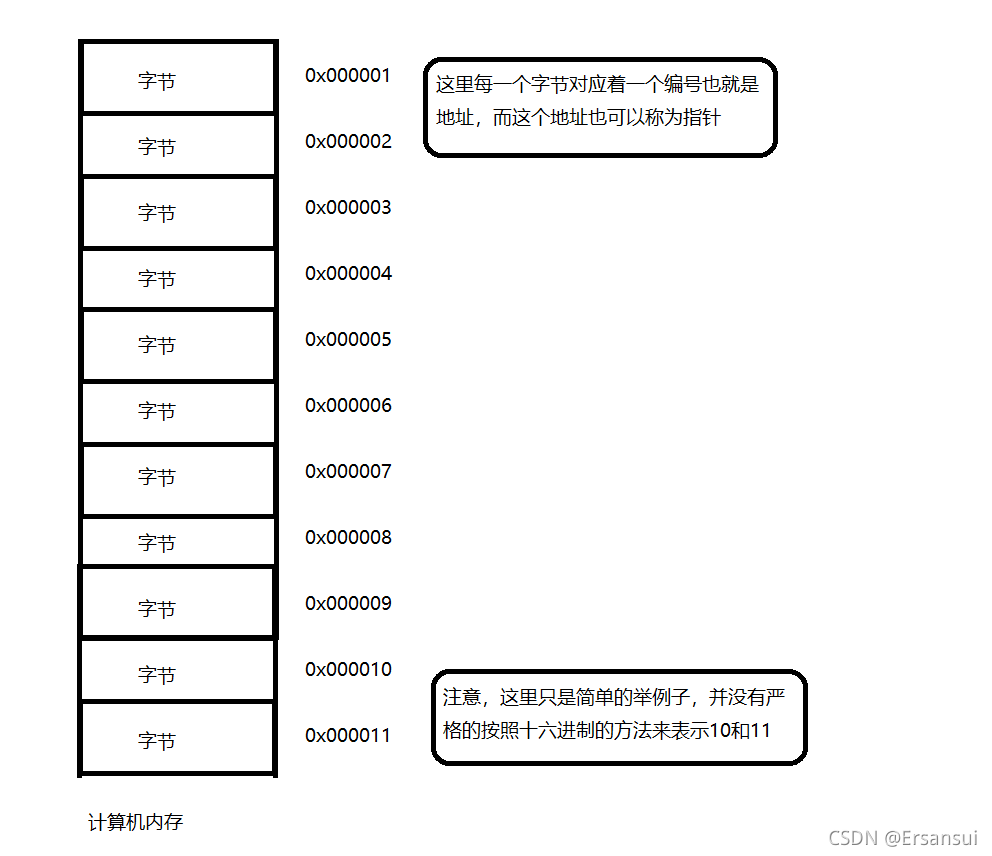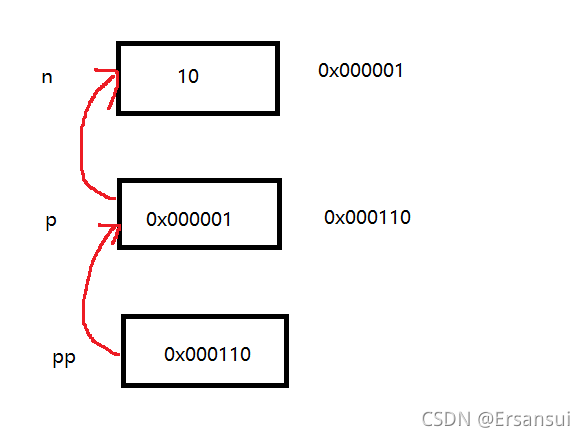# C | 指针的相关知识（一）int main()

{

int a = 10;//在内存中开辟一块整型空间

int* p;//这里的int* 是一种数据类型，用来定义了一个名为 p 的指针变量

p = &a;//&这个符号叫做取地址符，用来取出a变量的地址，并把这个地址赋值给了p

return 0;

}

int main()

{

int i = 0;

char c = 0;

short s = 0;

float f = 0;

double d = 0;

int* pi = &i;

char* pc = &c;

short* ps = &s;

float* pf = &f;

double* pd = &d;

return 0;

}

#include <stdio.h>

int main()

{

int* p;//局部变量指针未初始化，默认为随机值

*p = 20;

return 0;

}

#include <stdio.h>

int main()

{

int arr = { 0 };

int* p = arr;

int i = 0;

for (i = 0; i <= 11; i++)

{

//当指针指向的范围超出数组arr的范围时，p就是野指针

*(p++) = i;

}

return 0;

}

//检查指针的有效性

#include <stdio.h>

int main()

{

int *p = NULL;

//....

int a = 10;

p = &a;

if(p != NULL)

{

*p = 20;

}

return 0;

}

1.指针的类型决定了指针向前或者向后走一步有多大（距离）。

2.指针的类型决定了，对指针解引用的时候有多大的权限（能操作几个字节）。

（比如： char* 的指针解引用就只能访问一个字节，而 int* 的指针的解引用就能访问四个字节。）

#include <stdio.h>

//演示实例

int main()

{

int n = 10;

char *pc = (char*)&n;

int *pi = &n;

printf(%p\n, &n);

printf(%p\n, pc);

printf(%p\n, pc+1);

printf(%p\n, pi);

printf(%p\n, pi+1);

return  0;

}

int main()

{

int arr;

int* p1 = &arr;

int* p2 = &arr;

int n = p2 - p1;

printf(%d\n, n);

return 0;

}

sizeof（数组名）

&数组名int main()

{

int n = 10;

int* p = &n;

int** pp = &p;

return 0;

}

————————————————